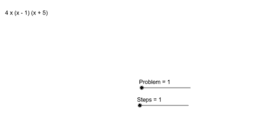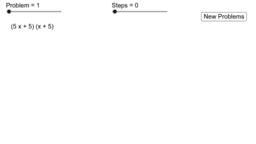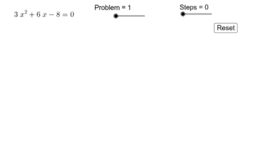Factoring

Author:
nnhsmath
There are three applets in this Geogebra book: Factoring 1: Factoring quadratic expressions with a lead coefficient of a = 1 Factoring 2: Factoring quadratic expressions with a lead coefficient of a <> 1 Factoring 3: Completing the Square Factoring 1, Factoring 2, and Completing the Square include a Steps slider which shows you the steps involved in factoring each expression. Hitting the refresh button will bring up a newly randomized problem.
•Factor1Rev

•Factor 2

•